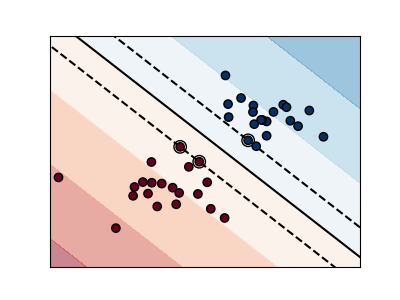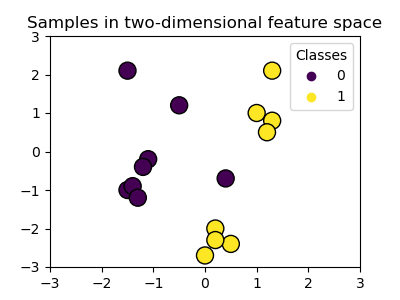# 监督学习：从高维数据中预测输出变量

scikit-learn中的所有有监督估计器都有一个拟合模型的`fit(X, y)`，和给定未标记的观察值`X`，返回预测的标记`y``predict(X)`方法。

## 最近邻分类器与维度惩罚

irises(鸢尾花)分类:iris(鸢尾花)数据集是一个分类任务，包括识别3种不同类型的鸢尾花(Setosa, Versicolour, and Virginica)的花瓣和萼片长度和宽度：

``````>>> import numpy as np
>>> from sklearn import datasets
>>> np.unique(iris_y)
array([0, 1, 2])
``````

### k近邻分类器

KNN (k近邻) 分类示例 :``````>>> # 将iris(鸢尾花)数据分成训练数据和测试数据
>>> # 随机排列，使得数据随机划分
>>> np.random.seed(0)
>>> indices = np.random.permutation(len(iris_X))
>>> iris_X_train = iris_X[indices[:-10]]
>>> iris_y_train = iris_y[indices[:-10]]
>>> iris_X_test = iris_X[indices[-10:]]
>>> iris_y_test = iris_y[indices[-10:]]
>>> # 创建和训练最近邻分类器
>>> from sklearn.neighbors import KNeighborsClassifier
>>> knn = KNeighborsClassifier()
>>> knn.fit(iris_X_train, iris_y_train)
KNeighborsClassifier()
>>> knn.predict(iris_X_test)
array([1, 2, 1, 0, 0, 0, 2, 1, 2, 0])
>>> iris_y_test
array([1, 1, 1, 0, 0, 0, 2, 1, 2, 0])
``````

## 线性模型: 从回归到稀疏

diabetes(糖尿病)数据集

diabetes(糖尿病)数据集由442位患者的10个生理变量（年龄，性别，体重，血压）组成，和一年后疾病级别指标：

``````>>> diabetes_X, diabetes_y = datasets.load_diabetes(return_X_y=True)
>>> diabetes_X_train = diabetes_X[:-20]
>>> diabetes_X_test  = diabetes_X[-20:]
>>> diabetes_y_train = diabetes_y[:-20]
>>> diabetes_y_test  = diabetes_y[-20:]
``````

`线性回归`，以其最简单拟合线性模型的形式，通过调整一系列参数使模型的残差平方和尽可能小。• X: 数据
• y: 目标变量
• β: 系数
• ε: 观测噪声
``````>>> from sklearn import linear_model
>>> regr = linear_model.LinearRegression()
>>> regr.fit(diabetes_X_train, diabetes_y_train)
LinearRegression()
>>> print(regr.coef_)
[   0.30349955 -237.63931533  510.53060544  327.73698041 -814.13170937
492.81458798  102.84845219  184.60648906  743.51961675   76.09517222]

>>> # 均方误差
>>> np.mean((regr.predict(diabetes_X_test) - diabetes_y_test)**2)
2004.56760268...

>>> # 解释方差得分：1为完美预测
>>> # 0表示X和y之间没有线性关系
>>> regr.score(diabetes_X_test, diabetes_y_test)
0.5850753022690...
``````

``````>>> X = np.c_[ .5, 1].T
>>> y = [.5, 1]
>>> test = np.c_[ 0, 2].T
>>> regr = linear_model.LinearRegression()

>>> import matplotlib.pyplot as plt
>>> plt.figure()

>>> np.random.seed(0)
>>> for _ in range(6):
...     this_X = .1 * np.random.normal(size=(2, 1)) + X
...     regr.fit(this_X, y)
...     plt.plot(test, regr.predict(test))
...     plt.scatter(this_X, y, s=3)
````````````>>> regr = linear_model.Ridge(alpha=.1)
>>> plt.figure()

>>> np.random.seed(0)
>>> for _ in range(6):
...     this_X = .1 * np.random.normal(size=(2, 1)) + X
...     regr.fit(this_X, y)
...     plt.plot(test, regr.predict(test))
...     plt.scatter(this_X, y, s=3)
``````

``````>>> alphas = np.logspace(-4, -1, 6)
>>> print([regr.set_params(alpha=alpha)
...            .fit(diabetes_X_train, diabetes_y_train)
...            .score(diabetes_X_test, diabetes_y_test)
...        for alpha in alphas])
[0.5851110683883..., 0.5852073015444..., 0.5854677540698...,
0.5855512036503..., 0.5830717085554..., 0.57058999437...]
``````

：捕获拟合参数的噪声（该噪声阻止模型将其推广到新数据）称为 过拟合。岭回归产生的偏差称为 正则化``````>>> regr = linear_model.Lasso()
>>> scores = [regr.set_params(alpha=alpha)
...               .fit(diabetes_X_train, diabetes_y_train)
...               .score(diabetes_X_test, diabetes_y_test)
...           for alpha in alphas]
>>> best_alpha = alphas[scores.index(max(scores))]
>>> regr.alpha = best_alpha
>>> regr.fit(diabetes_X_train, diabetes_y_train)
Lasso(alpha=0.025118864315095794)
>>> print(regr.coef_)
[   0.         -212.43764548  517.19478111  313.77959962 -160.8303982    -0.
-187.19554705   69.38229038  508.66011217   71.84239008]
``````y = sigmoid(X β - offset) + ε = 1 / (1 + exp(-X β + offset) + ε

``````>>> log = linear_model.LogisticRegression(C=1e5)
>>> log.fit(iris_X_train, iris_y_train)
LogisticRegression(C=100000.0)
``````C参数控制 逻辑回归(LogisticRegression) 对象中的正则化量：C值较大会导致正则化程度降低。`penalty = "l2"` 提供收缩率（即非稀疏系数），而 `penalty = "l1"` 提供稀疏率。

``````from sklearn import datasets, neighbors, linear_model

X_digits = X_digits / X_digits.max()
``````

## 支持向量机 (SVMs)

### 线性支持向量机``````>>> from sklearn import svm
>>> svc = svm.SVC(kernel='linear')
>>> svc.fit(iris_X_train, iris_y_train)
SVC(kernel='linear')
``````

### 使用核(kernels)``````>>> svc = svm.SVC(kernel='linear')
``````

``````>>> svc = svm.SVC(kernel='poly',degree=3)
>>> # degree: 多项式次数
``````

RBF 核 (径向基函数)

``````>>> svc = svm.SVC(kernel='rbf')
>>> # gamma:径向核大小的逆
````````````iris = datasets.load_iris()
X = iris.data
y = iris.target

X = X[y != 0, :2]
y = y[y != 0]
``````

(C) 2007 - 2019, scikit-learn 开发人员（BSD许可证）. 查看此页源代码